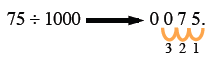### Home > CC1 > Chapter 5 > Lesson 5.1.4 > Problem5-38

5-38.

Divide each pair of numbers below. Homework Help ✎

1. $75 \div 10$

• $7.5$

1. $75 \div 100$

• $0.75$

1. $75 \div 1000$

• How many spaces do you need to move the decimal point? In what direction do you need to move the decimal point?

1. Describe in words what happens to the decimal point in each problem, (a) through (c) above.

• What is happening in each case? How is this related to what you have seen before?